Request a Free Counselling Session from our Expert Mentor# NCERT Solution for Class 12 Chemistry Chapter 9

Welcome to Swastik Classes’ NCERT Solutions for Class 12 Chemistry Chapter 9 – Coordination Compounds. This chapter is an important part of inorganic chemistry and deals with the study of coordination compounds. Coordination compounds are complex molecules consisting of a central metal ion and ligands attached to it.

Our NCERT Solutions for Class 12 Chemistry Chapter 9 aim to provide students with a comprehensive understanding of the concepts covered in the chapter. Our solutions are designed by subject matter experts with vast experience in the field of chemistry. We have provided step-by-step solutions to all the questions in the chapter, making it easy for students to understand and follow the concepts.

Our solutions also include illustrations and diagrams to help students visualize the concepts and remember them better. Additionally, we have included sample questions and answers to help students prepare for their exams.

With our NCERT Solutions for Class 12 Chemistry Chapter 9, students can gain a deeper understanding of coordination compounds, their nomenclature, isomerism, bonding, and various types of reactions. They can also score well in their exams and build a strong foundation in inorganic chemistry.

## Answers for chemistry class 12 chapter 9 Coordination Compound

### Chemistry Class 12 NCERT solutions chapter 9 intext questions and answers

Question 9.1:

Write the formulas for the following coordination compounds:

(i)  Tetraamminediaquacobalt(III) chloride

(ii)  Potassium tetracyanonickelate(II)

(iii)  Tris(ethane−1,2−diamine) chromium(III) chloride

(iv)  Amminebromidochloridonitrito-N-platinate(II)

(v)  Dichloridobis(ethane−1,2−diamine)platinum(IV) nitrate

(vi)  Iron(III) hexacyanoferrate(II)

(i)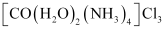(ii)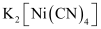(iii)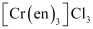(vi)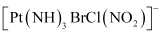(v)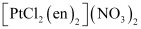(vi)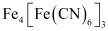Question 9.2:

Write the IUPAC names of the following coordination compounds:

(i)  [Co(NH3)6]Cl3

(ii)  [Co(NH3)5Cl]Cl2

(iii)  K3[Fe(CN)6]

(iv)  K3[Fe(C2O4)3]

(v)  K2[PdCl4]

(vi)  [Pt(NH3)2Cl(NH2CH3)]Cl

(i)  Hexaamminecobalt(III) chloride

(ii)  Pentaamminechloridocobalt(III) chloride

(iii)  Potassium hexacyanoferrate(III)

(iv)  Potassium trioxalatoferrate(III)

(vi) Diamminechlorido(methylamine)platinum(II) chloride

Question 9.3:

Indicate the types of isomerism exhibited by the following complexes and

draw the structures for these isomers:

1. K[Cr(H2O)2(C2O4)2
2. [Co(en)3]Cl3
3. [Co(NH3)5(NO2)](NO3)2
4. [Pt(NH3)(H2O)Cl2]

1. Both geometrical (cis-, trans-) isomers forK[Cr(H2O)2(C2O4)2can exist. Also, optical isomers for cis-isomer exist.

Trans-isomer is optically inactive. On the other hand, cis-isomer is optically active.

1. Two optical isomers for [Co(en)3]Cl3 exist.

Two optical isomers are possible for this structure.

1. [Co(NH3)5(NO2)](NO3)2

A pair of optical isomers:

It can also show linkage isomerism.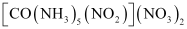and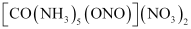It can also show ionization isomerism.

1. Geometrical (cis-trans-) isomers of [Pt(NH3)(H2O)Cl2] can exist.

Question 9.4:

Give evidence that [Co(NH3)5Cl]SO4 and [Co(NH3)5SO4]Cl are ionization isomers.

When ionization isomers are dissolved in water, they ionize to give different ions. These ions then react differently with different reagents to give different products.

Question 9.5:

Explain on the basis of valence bond theory that [Ni(CN)4]2− ion with square

planar structure is diamagnetic and the [NiCl4]2− ion with tetrahedral geometry is paramagnetic.

Ni is in the +2 oxidation state i.e., in d8 configuration.

There are 4 CN ions. Thus, it can either have a tetrahedral geometry or square planar geometry. Since CN ion is a strong field ligand, it causes the pairing of unpaired 3d electrons.

It now undergoes dsp2 hybridization. Since all electrons are paired, it is diamagnetic.

In case of [NiCl4]2−, Cl ion is a weak field ligand. Therefore, it does not lead to the pairing of unpaired 3d electrons. Therefore, it undergoes sp3 hybridization.

Since there are 2 unpaired electrons in this case, it is paramagnetic in nature.

Question 9.6:

[NiCl4]2− is paramagnetic while [Ni(CO)4] is diamagnetic though both are tetrahedral. Why?

Though both [NiCl4]2− and [Ni(CO)4] are tetrahedral, their magnetic characters are different. This is due to a difference in the nature of ligands. Cl is a weak field ligand and it does not cause the pairing of unpaired 3d electrons. Hence, [NiCl4]2− is paramagnetic.

In Ni(CO)4, Ni is in the zero oxidation state i.e., it has a configuration of 3d8 4s2.

But CO is a strong field ligand. Therefore, it causes the pairing of unpaired 3d electrons. Also, it causes the 4s electrons to shift to the 3d orbital, thereby giving rise to sp3 hybridization. Since no unpaired electrons are present in this case, [Ni(CO)4] is diamagnetic.

Question 9.7:

[Fe(H2O)6]3+ is strongly paramagnetic whereas [Fe(CN)6]3− is weakly paramagnetic. Explain.

In both [Fe(H2O)6]3+ and [Fe(CN)6]3- , Fe exists in the +3 oxidation state i.e., in d5 configuration.

Since CN is a strong field ligand, it causes the pairing of unpaired electrons. Therefore, there is only one unpaired electron left in the d-orbital.

Therefore,

On the other hand, H2O is a weak field ligand. Therefore, it cannot cause the pairing of electrons. This means that the number of unpaired electrons is 5.

Therefore,

Thus, it is evident that [Fe(H2O)6]3+ is strongly paramagnetic, while [Fe(CN)6]3- is weakly paramagnetic.

Question 9.8:

Explain [Co(NH3)6]3+ is an inner orbital complex whereas [Ni(NH3)6]2+ is an outer orbital complex.

Question 9.9:

Predict the number of unpaired electrons in the square planar [Pt(CN)4]2− ion.

In this complex, Pt is in the +2 state. It forms a square planar structure. This means that it undergoes dsp2 hybridization. Now, the electronic configuration of Pd(+2) is 5d8.

CN being a strong field ligand causes the pairing of unpaired electrons. Hence, there are no unpaired electrons in[Pt(CN)4]2−

Question 9.10:

The hexaquo manganese(II) ion contains five unpaired electrons, while the hexacyanoion contains only one unpaired electron. Explain using Crystal Field Theory.

Hence, hexaaquo manganese (II) ion has five unpaired electrons, while hexacyano ion has only one unpaired electron.

Question 9.11:

Calculate the overall complex dissociation equilibrium constant for the Cu(NH3)42+ ion, given that β4 for this complex is 2.1 × 1013.

β= 2.1 × 1013

The overall complex dissociation equilibrium constant is the reciprocal of the overall stability constant, β4.

### NCERT Class 12 Chemistry Chapter 9 Exercise Solutions

Question 9.1:

Explain the bonding in coordination compounds in terms of Werner’s postulates.

Werner’s postulates explain the bonding in coordination compounds as follows:

(i)  A metal exhibits two types of valences namely, primary and secondary valences. Primary valences are satisfied by negative ions while secondary valences are satisfied by both negative and neutral ions.

(In modern terminology, the primary valency corresponds to the oxidation number of the metal ion, whereas the secondary valency refers to the coordination number of the metal ion.

(ii)  A metal ion has a definite number of secondary valences around the central atom. Also, these valences project in a specific direction in the space assigned to the definite geometry of the coordination compound.

(iii)  Primary valences are usually ionizable, while secondary valences are non-ionizable.

*Question 9.2:

FeSO4 solution mixed with (NH4)2SO4 solution in 1:1 molar ratio gives the test of Fe2+ ion but CuSOsolution mixed with aqueous ammonia in 1:4 molar ratio does not give the test of Cu2+ ion. Explain why?

Both the compounds fall under the category of addition compounds with only one major difference i.e., the former is an example of a double salt, while the latter is a coordination compound.

A double salt is an addition compound that is stable in the solid state but that which breaks up into its constituent ions in the dissolved state. These compounds exhibit individual properties of their constituents. For e.g. FeSO4.(NH4)2.SO4.6H2O breaks into Fe2+, NH4+, and SO42− ions. Hence, it gives a positive test for Fe2+ ions.

A coordination compound is an addition compound which retains its identity in the solid as well as in the dissolved state. However, the individual properties of the constituents are lost. This happens because [Cu(NH3)4]SO4.5H2O does not show the test for Cu2+. The ions present in the solution of [Cu(NH3)4]SO4.5H2O are [Cu(NH3)4]+ and sulphate ions

*Question 9.3:

Explain with two examples each of the following: coordination entity, ligand, coordination number, coordination polyhedron, homoleptic and heteroleptic.

(i)  Coordination entity:

In a coordination entity, the central atom or ion is surrounded by a suitable number of neutral molecules or negative ions (called ligands). It can be positively or negatively charged as well as neutral also. For example: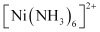= cationic complex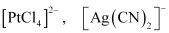= anionic complex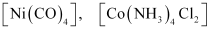= neutral complex

(ii)  Ligands

The neutral molecules or negatively charged ions that surround the metal atom in a coordination entity or a coordinal complex are known as ligands. For example: NH3, H2O, ClOH. Ligands are usually polar in nature and possess at least one unshared pair of valence electrons.

(iii) Coordination number:

The total number of ligands (either neutral molecules or negative ions) that get attached to the central metal atom in the coordination sphere is called the coordination number of the central metal atom. It is also referred to as its ligancy.

For example:

(a) In the complex, K2[PtCl6], there as six chloride ions attached to Pt in the coordinate sphere. Therefore, the coordination number of Pt is 6.

(b) Similarly, in the complex [Ni(NH3)4]Cl2, the coordination number of the central atom (Ni) is 4.

(vi)  Coordination polyhedron:

Coordination polyhedrons about the central atom can be defined as the spatial arrangement of the ligands that are directly attached to the central metal ion in the coordination sphere. For example:

(a)

(b) Tetrahedral

(v)  Homoleptic complexes:

These are those complexes in which the metal ion is bound to only one kind of a donor group. For eg.:

(vi)  Heteroleptic complexes:

Heteroleptic complexes are those complexes where the central metal ion is bound to more than one type of a donor group.For e.g.:

Question 9.4:

What is meant by unidentate, didentate and ambidentate ligands? Give two examples for each.

A ligand may contain one or more unshared pairs of electrons which are called the donor sites of ligands. Now, depending on the number of these donor sites, ligands can be classified as follows:

(a)  Unidentate ligands: Ligands with only one donor sites are called unidentate ligands. For e.g., NH3, Cl etc.

(b)  Didentate ligands: Ligands that have two donor sites are called didentate ligands. For e.g.,

(a) Ethane-1,2-diamine

(b) Oxalate ion

(c)  Ambidentate ligands:

Ligands that can attach themselves to the central metal atom through two different atoms are called ambidentate ligands. For example:

(a)

(The donor atom is N)

(The donor atom is oxygen)

(b)

(The donor atom is S)

(The donor atom is N)

Question 9.5:

Specify the oxidation numbers of the metals in the following coordination entities:

(i)  [Co(H2O)(CN)(en)2]2+

(ii)  [CoBr2(en)2]+

(iii)  [PtCl4]2−

(iv) K3[Fe(CN)6]

(v)  [Cr(NH3)3Cl3]

(i)  [Co(H2O)(CN)(en)2]2+

Let the oxidation number of Co be x.

The charge on the complex is +2.

(ii) [CoBr2(en)2]+

(iii)  [PtCl4]2−

Let the oxidation number of Pt be x.

The charge on the complex is −2.

x + 4(−1) = −2

x = + 2

(iv)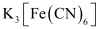(v)

*Question 9.6:

Using IUPAC norms write the formulas for the following:

(i)  Tetrahydroxozincate(II)

(iii)  Diamminedichloridoplatinum(II)

(iv)  Potassium tetracyanonickelate(II)

(v)  Pentaamminenitrito-O-cobalt(III)

(vi)  Hexaamminecobalt(III) sulphate

(vii)  Potassium tri(oxalato)chromate(III)

(viii)  Hexaammineplatinum(IV)

(ix)  Tetrabromidocuprate(II)

(x)  Pentaamminenitrito-N-cobalt(III)

(i)  [Zn(OH)4]2−

(ii)  K2[PdCl4]

(iii)  [Pt(NH3)2Cl2]

(iv)  K2[Ni(CN)4]

(v)  [Co(ONO) (NH3)5]2+

(vi)  [Co(NH3)6]2 (SO4)3

(vii)  K3[Cr(C2O4)3]

(viii)  [Pt(NH3)6]4+

(ix)  [Cu(Br)4]2−

(x)  [Co[NO2)(NH3)5]2+

*Question 9.7:

Using IUPAC norms write the systematic names of the following:

(i)  [Co(NH3)6]Cl3

(ii)  [Pt(NH3)2Cl(NH2CH3)]Cl

(iii)  [Ti(H2O)6]3+

(iv)  [Co(NH3)4Cl(NO2)]Cl

(v)  [Mn(H2O)6]2+

(vi)  [NiCl4]2−

(vii)  [Ni(NH3)6]Cl2

(viii)  [Co(en)3]3+

(ix)  [Ni(CO)4]

(i)  Hexaamminecobalt(III) chloride

(ii)  Diamminechlorido(methylamine) platinum(II) chloride

(iii)  Hexaquatitanium(III) ion

(iv)  Tetraamminichloridonitrito-N-Cobalt(III) chloride

(v)  Hexaquamanganese(II) ion

(vi)  Tetrachloridonickelate(II) ion

(vii)  Hexaamminenickel(II) chloride

(viii)  Tris(ethane-1, 2-diammine) cobalt(III) ion

(ix)  Tetracarbonylnickel(0)

Question 9.8:

List various types of isomerism possible for coordination compounds, giving an example of each.

(a)  Geometric isomerism:

This type of isomerism is common in heteroleptic complexes. It arises due to the different possible geometric arrangements of the ligands w.r.t. each other. For example:

(b)  Optical isomerism:

This type of isomerism arises in chiral molecules. Isomers are mirror images of each other and are non-superimposable.

(c)  Linkage isomerism: This type of isomerism is found in complexes that contain ambidentate ligands. For example:

[Co(NH3)5 (NO2)]Cl2 and [Co(NH3)5 (ONO)Cl2

(d)  Coordination isomerism:

This type of isomerism arises when the ligands are interchanged between cationic and anionic entities of differnet metal ions present in the complex.

[Co(NH3)6] [Cr(CN)6] and [Cr(NH3)6] [Co(CN)6]

(e)  Ionization isomerism:

This type of isomerism arises when a counter ion replaces a ligand within the coordination sphere. Thus, complexes that have the same composition, but furnish different ions when dissolved in water are called ionization isomers. For e.g., Co(NH3)5SO4)Br and Co(NH3)5Br]SO4.

(f)  Solvate isomerism:

Solvate isomers differ by whether or not the solvent molecule is directly bonded to the metal ion or merely present as a free solvent molecule in the crystal lattice. For ex:

[Cr[H2O)6]Cl3 [Cr(H2O)5Cl]Cl2⋅H2O [Cr(H2O)5Cl2]Cl⋅2H2O

Question 9.9:

How many geometrical isomers are possible in the following coordination entities?

(i)  [Cr(C2O4)3]3−  (ii) [Co(NH3)3Cl3]

(i)  For [Cr(C2O4)3]3−, no geometric isomer is possible as it is a bidentate ligand.

(ii)  [Co(NH3)3Cl3]

Two geometrical isomers are possible.

Question 9.10:

Draw the structures of optical isomers of:

(i)  [Cr(C2O4)3]3−

(ii)  [PtCl2(en)2]2+

(iii)  [Cr(NH3)2Cl2(en)]+

(i)  [Cr(C2O4)3]3−

(ii)  [PtCl2(en)2]2+

(iii)  [Cr(NH3)2Cl2(en)]+

Question 9.11:

Draw all the isomers (geometrical and optical) of:

(i)  [CoCl2(en)2]+

(ii)  [Co(NH3)Cl(en)2]2+

(iii) [Co(NH3)2Cl2(en)]+

(i)  [CoCl2(en)2]Geometrical isomerism

Optical isomerism Since only cis isomer is optically active, it shows optical isomerism.

In total, three isomers are possible.

(ii)  [Co(NH3)Cl(en)2]2+

Geometrical isomerism

Optical isomerism Since only cis isomer is optically active, it shows optical isomerism.

(iii)  [Co(NH3)2Cl2(en)]+

First isomer is cis-form and hence optically active; other two isomers are trans forms w.r.t. Cl and NH3. and hence optically inactive. First isomer exist as enatiomeric pair.

Question 9.12:

Write all the geometrical isomers of [Pt(NH3)(Br)(Cl)(py)] and how many of these will exhibit optical isomers?

[Pt(NH3)(Br)(Cl)(py)

From the above isomers, none will exhibit optical isomers as all of them have axis of symmetry.

*Question 9.13:

Aqueous copper sulphate solution (blue in colour) gives:

(i)  a green precipitate with aqueous potassium fluoride, and

(ii)  a bright green solution with aqueous potassium chloride

Explain these experimental results.

Aqueous CuSO4 exists as [Cu(H2O)4]SO4. It is blue in colour due to the presence of[Cu[H2O)4]2+ ions.

In both these cases, the weak field ligand water is replaced by the F and Cl ions.

Question 9.14:

What is the coordination entity formed when excess of aqueous KCN is added to an aqueous solution of copper sulphate? Why is it that no precipitate of copper sulphide is obtained when H2S(g) is passed through this solution?

i.e.,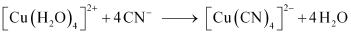Thus, the coordination entity formed in the process isK2[Cu(CN)4].

K2[Cu(CN)4] is a very stable complex, which does not ionize to give Cu2+ ions when added to water. Hence, Cu2+ ions are not precipitated when H2S(g) is passed through the solution.

*Question 9.15:

Discuss the nature of bonding in the following coordination entities on the basis of valence bond theory:

(i)  [Fe(CN)6]4−

(ii)  [FeF6]3−

(iii)  [Co(C2O4)3]3−

(iv)  [CoF6]3−

(i)  [Fe(CN)6]4−

In the above coordination complex, iron exists in the +II oxidation state.

Fe2+: Electronic configuration is 3d6

Orbitals of Fe2+ ion:

As CN is a strong field ligand, it causes the pairing of the unpaired 3d electrons.

Since there are six ligands around the central metal ion, the most feasible hybridization is d2sp3.

d2sphybridized orbitals of Fe2+ are:

6 electron pairs from CN ions occupy the six hybrid d2sp3orbitals.

Then,

Hence, the geometry of the complex is octahedral, and the complex is diamagnetic (as there are no unpaired electrons).

(ii)  [FeF6]3−

In this complex, the oxidation state of Fe is +3.

Orbitals of Fe+3 ion:

There are 6 F ions. Thus, it will undergo d2spor sp3d2 hybridization. As F− is a weak field ligand, it does not cause the pairing of the electrons in the 3d orbital. Hence, the most feasible hybridization is sp3d2.

sp3d2 hybridized orbitals of Fe are:

Hence, the geometry of the complex is found to be octahedral.

(iii)  [Co(C2O4)3]3−

Cobalt exists in the +3 oxidation state in the given complex.

Orbitals of Co3+ ion:

Oxalate is a strong field ligand. Therefore, it can cause the pairing of the 3orbital electrons. As there are 6 ligands, hybridization has to be either sp3d2 or d2sp3 hybridization.

However due to pairing; two inner d-orbitals are vacant and hence hybridization is d2sp3

Hence, the geometry of the complex is found to be octahedral.

(iv)  [CoF6]3−

Cobalt exists in the +3 oxidation state.

Orbitals of Co3+ ion:

Again, fluoride ion is a weak field ligand. It cannot cause the pairing of the 3d electrons. As a result, the Co3+ ion will undergo sp3d2 hybridization.

sp3d2 hybridized orbitals of Co3+ ion are:

Hence, the geometry of the complex is octahedral and paramagnetic.

Question 9.16:

Draw figure to show the splitting of orbitals in an octahedral crystal field.

The splitting of the d orbitals in an octahedral field takes palce in such a way that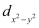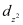experience a rise in energy and form the eg level, while dxy, dyzand dzx experience a fall in energy and form the t2g level.

Question 9.17:

What is spectrochemical series? Explain the difference between a weak field ligand and a strong field ligand.

A spectrochemical series is the arrangement of common ligands in the increasing order of their crystal-field splitting energy (CFSE) values. The ligands present on the R.H.S of the series are strong field ligands while that on the L.H.S are weak field ligands. Also, strong field ligands cause higher splitting in the d orbitals than weak field ligands.

I− < Br < SCN < Cl<S2−< F < OH < oxalate < H2O < NCS < edta <NH3< en<CN< CO

Question 9.18:

What is crystal field splitting energy? How does the magnitude of Δo decide the actual configuration of d-orbitals in a coordination entity?

The degenerate d-orbitals (in a spherical field environment) split into two levels i.e., eg and t2g in the presence of ligands. The splitting of the degenerate levels due to the presence of ligands is called the crystal-field splitting while the energy difference between the two levels (eg and t2g) is called the crystal-field splitting energy. It is denoted by Δo.

After the orbitals have split, the filling of the electrons takes place. After 1 electron (each) has been filled in the three t2g orbitals, the filling of the fourth electron takes place in two ways. It can enter the eg orbital (giving rise to t2g3 eg1 like electronic configuration) or the pairing of the electrons can take place in the t2g orbitals (giving rise to t2g4 eg0 like electronic configuration). If the Δo value of a ligand is less than the pairing energy (P), then the electrons enter the eg orbital. On the other hand, if the Δo value of a ligand is more than the pairing energy (P), then the electrons enter the t2g orbital.

Question 9.19:

[Cr(NH3)6]3+ is paramagnetic while [Ni(CN)4]2− is diamagnetic. Explain why?

Cr is in the +3 oxidation state i.e., d3 configuration. So no need of pairing is there.

Cr3+

Therefore, it undergoes d2sp3 hybridization and the electrons in the 3d orbitals remain unpaired. Hence, it is paramagnetic in nature.

In [Ni(CN)4]2−, Ni exists in the +2 oxidation state i.e., d8 configuration.

Ni2+:

CN is a strong field ligand. It causes the pairing of the 3d orbital electrons. Then, Ni2+ undergoes dsp2 hybridization.

As there are no unpaired electrons, it is diamagnetic.

Question 9.20:

A solution of [Ni(H2O)6]2+ is green but a solution of [Ni(CN)4]2− is colourless. Explain.

In [Ni(H2O)6]2+, water is a weak field ligand. Therefore, there are unpaired electrons in Ni2+. In this complex, the d electrons from the lower energy level can be excited to the higher energy level i.e., the possibility of d−d transition is present. Hence, Ni(H2O)6]2+ is coloured.

In [Ni(CN)4]2−, the electrons are all paired as CN is a strong field ligand. Therefore, d-d transition is not possible in [Ni(CN)4]2−. Hence, it is colourless.

Question 9.21:

[Fe(CN)6]4− and [Fe(H2O)6]2+ are of different colours in dilute solutions. Why?

The colour of a particular coordination compound depends on the magnitude of the crystal-field splitting energy, Δ. This CFSE in turn depends on the nature of the ligand. In case of [Fe(CN)6]4− and [Fe(H2O)6]2+, the colour differs because there is a difference in the CFSE. Now, CN is a strong field ligand having a higher CFSE value as compared to the CFSE value of water. This means that the absorption of energy for the intra d-d transition also differs. Hence, the transmitted colour also differs.

*Question 9.22:

Discuss the nature of bonding in metal carbonyls.

The metal-carbon bonds in metal carbonyls have both σ and π characters. A σ bond is formed when the carbonyl carbon donates a lone pair of electrons to the vacant orbital of the metal. A π bond is formed by the donation of a pair of electrons from the filled metal d orbital into the vacant anti-bonding π* orbital (also known as back bonding of the carbonyl group). The σ bond strengthens the π bond and vice-versa. Thus, a synergic effect is created due to this metal-ligand bonding. This synergic effect strengthens the bond between CO and the metal.

*Question 9.23:

Give the oxidation state, d-orbital occupation and coordination number of the central metal ion in the following complexes:

(i)  K3[Co(C2O4)3]

(ii)  cis-[Cr(en)2Cl2]Cl

(iii)  (NH4)2[CoF4]

(iv)  [Mn(H2O)6]SO4

(i)  K3[Co(C2O4)3]

The central metal ion is Co.

Its coordination number is 6.

The oxidation state can be given as:

x − 6 = −3

x = + 3

The d orbital occupation for Co3+ is t2g6eg0.

(ii)  cis-[Cr(en)2Cl2]Cl

The central metal ion is Cr.

The coordination number is 6.

The oxidation state can be given as:

+ 2(0) + 2(−1) = +1

x − 2 = +1

x = +3

The d orbital occupation for Cr3+ is t2g3.

(iii)  (NH4)2[CoF4]

The central metal ion is Co.

The coordination number is 4.

The oxidation state can be given as:

x − 4 = −2

x = + 2

The d orbital occupation for Co2+ is eg4 t2g3.

(iv)  [Mn(H2O)6]SO4

The central metal ion is Mn.

The coordination number is 6.

The oxidation state can be given as:

x + 0 = +2

x = +2

The d orbital occupation for Mn is t2g3 eg2.

*Question 9.24:

Write down the IUPAC name for each of the following complexes and indicate the oxidation state, electronic configuration and coordination number. Also give stereochemistry and magnetic moment of the complex:

(i)  K[Cr(H2O)2(C2O4)2].3H2O

(ii)  [Co(NH3)5Cl]Cl2

(iii)  CrCl3(py)3

(iv) Cs[FeCl4]

(v)  K4[Mn(CN)6]

Oxidation state of chromium = 3

Electronic configuration: 3d3t2g3

Coordination number = 6

Shape: octahedral

Stereochemistry:

Magnetic moment, μ

∼ 4BM

(ii) [Co(NH3)5Cl]Cl2

IUPAC name: Pentaamminechloridocobalt(III) chloride

Oxidation state of Co = +3

Coordination number = 6

Shape: octahedral.

Electronic configuration: d6t2g6.

Stereochemistry:

Magnetic Moment = 0

(iii) CrCl3(py)3

IUPAC name: Trichloridotripyridinechromium (III)

Oxidation state of chromium = +3

Electronic configuration for d3 = t2g3

Coordination number = 6

Shape: octahedral.

Stereochemistry:

Facial is optically active, meridional is optically inactive.

Magnetic moment, μ

∼ 4BM

(iv) Cs[FeCl4]

IUPAC name: Caesium tetrachloroferrate (III)

Oxidation state of Fe = +3

Electronic configuration of d6 = eg2t2g3

Coordination number = 4

Shape: tetrahedral

Stereochemistry: optically inactive

Magnetic moment:

(v) K4[Mn(CN)6]

Potassium hexacyanomanganate(II)

Oxidation state of manganese = +2

Electronic configuration: d5+t2g5

Coordination number = 6

Shape: octahedral.

Streochemistry: optically inactive

Magnetic moment, μ

*Question 9.25:

What is meant by stability of a coordination compound in solution? State the factors which govern stability of complexes.

The stability of a complex in a solution refers to the degree of association between the two species involved in a state of equilibrium. Stability can be expressed quantitatively in terms of stability constant or formation constant.

For this reaction, the greater the value of the stability constant, the greater is the proportion of ML3 in the solution.

Stability can be of two types:

(a)  Thermodynamic stability:

The extent to which the complex will be formed or will be transformed into another species at the point of equilibrium is determined by thermodynamic stability.

(b)  Kinetic stability:

This helps in determining the speed with which the transformation will occur to attain the state of equilibrium.

Factors that affect the stability of a complex are:

(a)  Charge on the central metal ion: Greater the charge on the central metal ion, the greater is the stability of the complex.

(b) Basic nature of the ligand: A more basic ligand will form a more stable complex.

(c) Presence of chelate rings: Chelation increases the stability of complexes.

*Question 9.26:

What is meant by the chelate effect? Give an example.

When a ligand attaches to the metal ion in a manner that forms a ring, then the metal- ligand association is found to be more stable. In other words, we can say that complexes containing chelate rings are more stable than complexes without rings. This is known as the chelate effect.

For example:

*Question 9.27:

Discuss briefly giving an example in each case the role of coordination compounds in:

(i) biological system

(ii) medicinal chemistry

(iii) analytical chemistry

(iv) extraction/metallurgy of metals

(i) Role of coordination compounds in biological systems:

We know that photosynthesis is made possible by the presence of the chlorophyll pigment. This pigment is a coordination compound of magnesium. In the human biological system, several coordination compounds play important roles. For example, the oxygen-carrier of blood, i.e., haemoglobin, is a coordination compound of iron.

(ii)  Role of coordination compounds in medicinal chemistry:

Certain coordination compounds of platinum (for example, cis-platin) are used for inhibiting the growth of tumours.

(iii)  Role of coordination compounds in analytical chemistry:

During salt analysis, a number of basic radicals are detected with the help of the colour changes they exhibit with different reagents. These colour changes are a result of the coordination compounds or complexes that the basic radicals form with different ligands.

(iii)  Role of coordination compounds in extraction or metallurgy of metals:

The process of extraction of some of the metals from their ores involves the formation of complexes. For example, in aqueous solution, gold combines with cyanide ions to form [Au(CN)2]. From this solution, gold is later extracted by the addition of zinc metal.

Question 9.28:

How many ions are produced from the complex Co(NH3)6Cl2 in solution?

(i)  6

(ii) 4

(iii)  3

(iv)  2

(iii)  The given complex can be written as [Co(NH3)6]Cl2.

Thus[Co(NH3)6]+ along with two Cl− ions are produced.

*Question 9.29:

Amongst the following ions which one has the highest magnetic moment value?

(i)  [Cr(H2O)6]3+

(ii)  [Fe(H2O)6]2+

(iii)  [Zn(H2O)6]2+

(i)  No. of unpaired electrons in [Cr(H2O)6]3+ = 3

Then, μ

(ii)  No. of unpaired electrons in [Fe(H2O)6]2+ = 4

Then, μ

(iii)  No. of unpaired electrons in [Zn(H2O)6]2+ = 0

Hence, [Fe(H2O)6]2+ has the highest magnetic moment value.

Question 9.30:

The oxidation number of cobalt in K[Co(CO)4] is

(i)  +1

(ii)  +3

(iii)  −1

(iv)  −3

(iii) We know that CO is a neutral ligand and K carries a charge of +1.

Therefore, the complex can be written as K+[Co(CO)4]. Therefore, the oxidation number of Co in the given complex is −1. Hence, option (iii) is correct.

*Question 9.31:

Amongst the following, the most stable complex is

(i)  [Fe(H2O)6]3+

(ii)  [Fe(NH3)6]3+

(iii)  [Fe(C2O4)3]3−

(iv)  [FeCl6]3−

We know that the stability of a complex increases by chelation. Therefore, the most stable complex is [Fe(C2O4)3]3−.

Question 9.32:

What will be the correct order for the wavelengths of absorption in the visible region for the following:

[Ni(NO2)6]4−, [Ni(NH3)6]2+, [Ni(H2O)6]2+

The central metal ion in all the three complexes is the same. Therefore, absorption in the visible region depends on the ligands. The order in which the CFSE values of the ligands increases in the spectrochemical series is as follows:

H2O < NH3 < NO2

Thus, the amount of crystal-field splitting observed will be in the following order:

Hence, the wavelengths of absorption in the visible region will be in the order:

[Ni(H2O)6]2+ > [Ni(NH3)6]2+ > [Ni(NO2)6]4−

## Topics to study in Chemistry class 12 chapter 9

 Section Number Topics .9.1 Werner’s Theory of Coordination Compounds 9.2 Definitions of Some Important Terms Pertaining to Coordination Compounds 9.3 Nomenclature of Coordination Compounds 9.4 Isomerism in Coordination Compounds 9.5 Bonding in Coordination Compounds 9.6 Bonding in Metal Carbonyls 9.7 Stability of Coordination Compounds 9.8 Importance and Applications of Coordination Compounds

## Weightage of chemistry class 12 chapter 9

This chapter is going to give you 5 marks in your CBSE Term I exam. You can grab all 5 marks by practicing questions and learning concepts. By this, you will be able to solve the objective types of questions in your exam in a very short period of time.

 Chapter Marks Coordination Compound 5 Marks

## Why Opt for SWC?

At SWC, students directly learn from IITians and experienced faculties. We provide quality study materials to prepare and follow a LEAP model. We teach not only for exams but for lifelong applications## What are the types of Structural Isomerism?## Conclusion

Swastik Classes’ NCERT Solutions for Class 12 Chemistry Chapter 9 – Coordination Compounds provide a comprehensive understanding of the concepts covered in the chapter. The chapter deals with the study of coordination compounds, which are complex molecules consisting of a central metal ion and ligands attached to it.

Our solutions are designed by subject matter experts with vast experience in the field of chemistry. We have provided step-by-step solutions to all the questions in the chapter, which help students understand and follow the concepts easily. Our solutions also include illustrations and diagrams to help students visualize the concepts and remember them better.

By using our NCERT Solutions for Class 12 Chemistry Chapter 9, students can gain a deeper understanding of coordination compounds, their nomenclature, isomerism, bonding, and various types of reactions. Additionally, our solutions include sample questions and answers to help students prepare for their exams and build a strong foundation in inorganic chemistry.

Overall, our NCERT Solutions for Class 12 Chemistry Chapter 9 – Coordination Compounds are an effective and comprehensive resource for students to learn and excel in the subject.

## FAQs on NCERT Class 12 Chemistry Chapter 9

### What is a coordination compound with example?

In the coordination compound, the central atom or ion is bonded with the other groups of atoms or ions by coordinate bonds. These coordinate bonds are also known as coordination complexes. The atoms or ions which bond with the central atom are called Ligands or complexing agents.

Examples of coordination compounds are Chlorophyll, Haemoglobin, dyes, Vitamin B12, etc.

### What kind of questions can I expect from Chapter 9 of NCERT Solutions for Class 12 Chemistry in the board exams?

Following are the several questions that you can expect from coordination compounds:

1. What are examples of Linkage isomerism?
2. What do you mean by the chelate effect?
3. How would you decide the stability of the coordination compound in solution?
4. Write the formula of the following compounds using IUPAC norms:
1. Sodium dicyanidoaurate (I)
2. Tetraamminechloridonitrito-N-platinum (IV) sulphate
5. Explain the following terms:
1. Ambidentate ligand
2. Crystal field splitting